# 支持向量机SVM（Support Vector Machine）¶

## 0. Explain SVM like I am a 5 year old¶## 1. 支持向量机SVM的原理¶

SVM（Support Vector Machines）支持向量机是在所有知名的数据挖掘及传统机器学习算法中最健壮，最准确的方法之一，它属于二分类算法，可以支持线性和非线性的分类。

### 1.1 基本原理¶【重点】支持向量机的核心任务是：最大化类间距，最小化类内距。

### 1.3 SVM的核函数¶

#### 1.3.1 为什么要使用核函数¶1. 在低维空间中完成特征计算
2. 通过核函数将输入空间中的特征映射到高维特征空间
3. 在高维特征空间中构造最优分离超平面

1. SVM的春天在于核函数
2. SVM不仅仅能用于二分类，也同样可以用于多分类；同时也可以实现回归和聚类；
3. 核函数的主要功能是将特征映射投射到高维空间以实现"线性"可分;
4. 相比于简单地将特征映射到高维空间，核函数的价值在于：它所有的计算都是基于原始空间，只是将实质的分类效果表现到高维空间。这种机制避免的维度爬升的不可预见性（维度灾难），以及高维空间计算的复杂性。

#### 1.3.2 核函数的基本用法¶

• 线性核

1. 导入必须库
2. 创建/导入数据
3. 数据预处理，包括训练集、测试集划分，数据正则化，数据清洗等
4. 构建模型，并进行模型训练（或称为拟合数据）
5. 输出模型准确率
6. 可视化分析
In :
# 1.导入必须库
import numpy as np
import matplotlib.pyplot as plt
# 导入支持向量机SVM
from sklearn import svm
# 导入数据集生成和拆分工具
from sklearn.datasets import make_blobs
from sklearn.model_selection import train_test_split

# 2.创建/导入数据
X, y = make_blobs(n_samples=100, centers=2, random_state=6)

# 3.数据预处理，包括训练集、测试集划分，数据正则化，数据清洗等
X_train, X_test, y_train, y_test = train_test_split(X, y)

# 4.构建模型，并进行模型训练（或称为拟合数据）
clf = svm.SVC(kernel='linear', C=1000)
clf.fit(X_train, y_train)

# 5.输出模型准确率
print("线性SVM训练集评分为: {0:.3f}, 测试集评分为: {1:.3f}".format(clf.score(X_train, y_train), clf.score(X_test, y_test)))

# 6.可视化分析
# c: 色彩或颜色序列; s: 散点尺寸; cmap: Colormap
plt.scatter(X_train[:,0], X_train[:,1], c=y_train, s=30, cmap=plt.cm.Paired)

# 建立图像坐标
ax = plt.gca()
xlim = ax.get_xlim()
ylim = ax.get_ylim()

# 生成两个等差数列
xx = np.linspace(xlim, xlim, 30)
yy = np.linspace(ylim, ylim, 30)
YY, XX = np.meshgrid(yy, xx) # 生成网格采样点
xy = np.vstack([XX.ravel(), YY.ravel()]).T
Z = clf.decision_function(xy).reshape(XX.shape)

# 绘制分类的决定边界
ax.contour(XX, YY, Z, colors='k', levels=[-1, 0, 1], alpha=0.5, linestyles=['--', '-', '--'])
# 加强绘制支持向量
ax.scatter(clf.support_vectors_[:,0], clf.support_vectors_[:,1], s=100, linewidth=1, facecolors='None')

plt.savefig(r'results/Ch0801LinearSVM.png', dpi=300)
plt.show()

线性SVM训练集评分为: 1.000, 测试集评分为: 1.000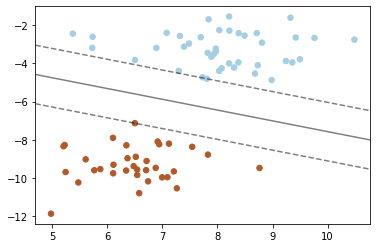【结果分析】从上图中可以看到, 两条虚线分别位于实线的两侧, 同时经过了至少一个样本数据点. 此时,我们可以将这些落在虚线上的样本称之为支持向量. 在上面的例子中, 我们使用的是SVM中最基本的"最大边界间隔超平面", 它使得图中的实线与所有支持向量之间的距离, 加权和最大. 下面我们尝试使用RBF核函数替代Linear核函数.

• RBF核

RBF核又称为高斯核,它的数学表达为: $k_{rbf}(x_1, x_2) = exp(\gamma ||x_1 - x_2||^2)$. 公式中,$\gamma$是用来控制RBF核宽度参数, 也就是"超平面"与"支持向量"所在虚线之间的距离; $x_1, x_2$分别代表不同的数据点, $||x_1 - x_2||$代表两点之间的欧几里得距离.

【知识点】 在二维的笛卡尔坐标系中, 点A($x_1, y_1$)和点B($x_2, y_2$)之间的欧几里得距离(欧氏距离) $D=\sqrt{(x_2-x_1)^2 + (y_2-y_1)^2}$.

In :
# 1.导入必须库
import numpy as np
import matplotlib.pyplot as plt
# 导入支持向量机SVM
from sklearn import svm
# 导入数据集生成和拆分工具
from sklearn.datasets import make_blobs
from sklearn.model_selection import train_test_split

# 2.创建/导入数据
X, y = make_blobs(n_samples=100, centers=2, random_state=6)

# 3.数据预处理，包括训练集、测试集划分，数据正则化，数据清洗等
X_train, X_test, y_train, y_test = train_test_split(X, y)

# 4.构建模型，并进行模型训练（或称为拟合数据）
clf_rbf = svm.SVC(kernel='rbf', C=1000, gamma='auto')
clf_rbf.fit(X_train, y_train)

# 5.输出模型准确率
print("线性SVM训练集评分为: {0:.3f}, 测试集评分为: {1:.3f}".format(clf.score(X_train, y_train), clf.score(X_test, y_test)))

# 6.可视化分析
# c: 色彩或颜色序列; s: 散点尺寸; cmap: Colormap
plt.scatter(X_train[:,0], X_train[:,1], c=y_train, s=30, cmap=plt.cm.Paired)

# 建立图像坐标
ax = plt.gca()
xlim = ax.get_xlim()
ylim = ax.get_ylim()

# 生成两个等差数列
xx = np.linspace(xlim, xlim, 30)
yy = np.linspace(ylim, ylim, 30)
YY, XX = np.meshgrid(yy, xx)
xy = np.vstack([XX.ravel(), YY.ravel()]).T
Z = clf_rbf.decision_function(xy).reshape(XX.shape)

# 绘制分类的决定边界
ax.contour(XX, YY, Z, colors='k', levels=[-1, 0, 1], alpha=0.5, linestyles=['--', '-', '--'])
# 加强绘制支持向量
ax.scatter(clf_rbf.support_vectors_[:,0], clf_rbf.support_vectors_[:,1], s=100, linewidth=1, facecolors='b')

plt.savefig(r'results/Ch0801LinearSVM.png', dpi=300)
plt.show()

线性SVM训练集评分为: 1.000, 测试集评分为: 1.000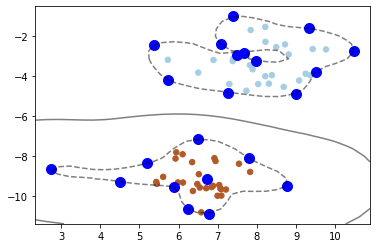## 2. SVM核函数和参数选择¶

### 2.1 基于不同核函数的SVM的对比¶

In :
# TODO: 1. 导入必须库 以及 定义必要的函数
import numpy as np
import matplotlib.pyplot as plt
# 导入 Wine酒 数据集
from sklearn import datasets
from sklearn.model_selection import train_test_split
# 导入支持向量机SVM
from sklearn import svm

# 定义一个绘图函数

def make_meshgrid(x, y, h=0.02):
x_min, x_max = x.min() - 1, x.max() - 1
y_min, y_max = y.min() - 1, y.max() - 1
xx, yy = np.meshgrid(np.arange(x_min, x_max, h),
np.arange(y_min, y_max, h))
return xx, yy

# 第一个一个绘制等高线的函数

def plot_contours(ax, clf, xx, yy, **params):
Z = clf.predict(np.c_[xx.ravel(), yy.ravel()])
Z = Z.reshape(xx.shape)
out = ax.contourf(xx, yy, Z, **params)
return out

# TODO: 2. 创建/导入数据

# TODO: 3. 数据预处理，包括训练集、测试集划分，数据正则化，数据清洗等
X = wine.data[:, :2]  # 为便于可视化仍然仅使用前两个特征
y = wine.target
X_train, X_test, y_train, y_test = train_test_split(X, y)

# TODO: 4. 构建模型，并进行模型训练（或称为拟合数据）
C = 1.0
models = (svm.LinearSVC(C=C, max_iter=10000),
svm.SVC(kernel='linear', C=C, gamma='auto'),
svm.SVC(kernel='rbf', gamma=0.7, C=C),
svm.SVC(kernel='sigmoid', C=C, gamma='auto'),
svm.SVC(kernel='poly', degree=3, C=C, gamma='auto'),
svm.SVC(kernel='poly', degree=4, C=C, gamma='auto'))
# TODO:考虑输出变量models
models = (clf.fit(X_train, y_train) for clf in models)

titles = ('LinearSVC (linear kernel)',
'SVC with linear kernel',
'SVC with RBF kernel',
'SVC with sigmoid kernel',
'SVC with ploy(degree=3) kernel',
'SVC with ploy(degree=4) kernel')

# TODO: 5. 输出模型准确率
# for model, title in zip(models, titles):
#     print("模型名称: {0}, 训练集准确率: {1:.3f}, 测试集准确率: {2:.3f}".format(
#         title, model.score(X_train, y_train), model.score(X_test, y_test)))

# TODO: 6. 可视化分析
# 设定一个子图形的个数和排列方式
fig, sub = plt.subplots(3, 2, figsize=(10, 10))

# 使用自定义的函数进行画图
X0, X1 = X_train[:, 0], X_train[:, 1]
xx, yy = make_meshgrid(X0, X1)

for clf, title, ax in zip(models, titles, sub.flatten()):
plot_contours(ax, clf, xx, yy, cmap=plt.cm.plasma, alpha=0.8)
ax.scatter(X0, X1, c=y_train, cmap=plt.cm.plasma, s=20, edgecolors='k')
ax.set_xlim(xx.min(), xx.max())
ax.set_ylim(yy.min(), yy.max())
ax.set_xlabel('Feature 0')
ax.set_ylabel('Feature 1')
ax.set_xticks(())
ax.set_yticks(())
ax.set_title(title)

# 输出准确率
print("模型名称: {0}, 训练集准确率: {1:.3f}, 测试集准确率: {2:.3f}".format(
title, clf.score(X_train, y_train), clf.score(X_test, y_test)))

plt.show()

模型名称: LinearSVC (linear kernel), 训练集准确率: 0.782, 测试集准确率: 0.667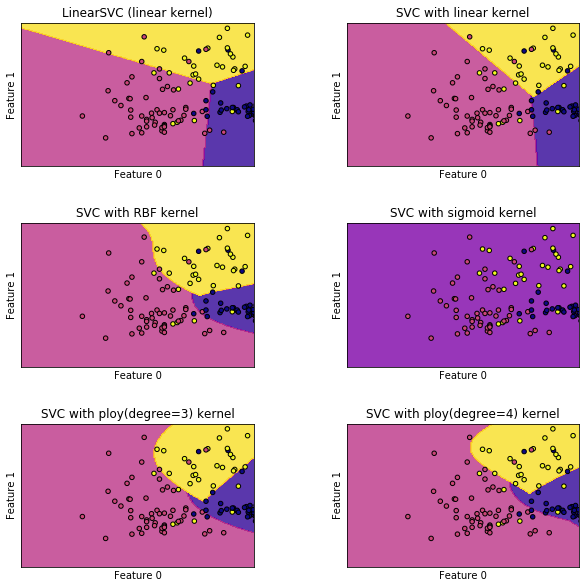【结果分析】

1. LinearSVC 和 SVC with linear核，由于都属于线性方法，所以性能相差不大，细微的差别主要来源于两种方法的正则化算法。linearSVC使用最小化L2范数，线性内核SVC使用最小化L1范数
2. 高斯核的RBF多项式核性能略微好一点，通过更具有弹性的非线性算法，它们能更好地拟合数据
3. Sigmoid核的SVM在这个任务中可以说是惨不忍睹，但是Sigmoid确是神经网络的一种重要激活函数
4. 超参数degree, 正则化参数C对基于多项式核的SVM具有较大影响
5. 超参数gamma, 正则化参数C对 基于高斯核(RBF核)的SVM具有较大影响

### 2.2 支持向量机的超参数调节与分析¶

#### 2.2.1 RBF核的gamma值¶

In :
# TODO: 1. 导入必须库 以及 定义必要的函数
import numpy as np
import matplotlib.pyplot as plt
# 导入 Wine酒 数据集
from sklearn import datasets
from sklearn.model_selection import train_test_split
# 导入支持向量机SVM
from sklearn import svm

# 定义一个绘图函数

def make_meshgrid(x, y, h=0.02):
x_min, x_max = x.min() - 1, x.max() - 1
y_min, y_max = y.min() - 1, y.max() - 1
xx, yy = np.meshgrid(np.arange(x_min, x_max, h),
np.arange(y_min, y_max, h))
return xx, yy

# 第一个一个绘制等高线的函数

def plot_contours(ax, clf, xx, yy, **params):
Z = clf.predict(np.c_[xx.ravel(), yy.ravel()])
Z = Z.reshape(xx.shape)
out = ax.contourf(xx, yy, Z, **params)
return out

# TODO: 2. 创建/导入数据

# TODO: 3. 数据预处理，包括训练集、测试集划分，数据正则化，数据清洗等
X = wine.data[:, :2]  # 为便于可视化仍然仅使用前两个特征
y = wine.target
X_train, X_test, y_train, y_test = train_test_split(X, y)

# TODO: 4. 构建模型，并进行模型训练（或称为拟合数据）
C = 1.0
models = (svm.SVC(kernel='rbf', gamma=0.1, C=C),
svm.SVC(kernel='rbf', gamma=1, C=C),
svm.SVC(kernel='rbf', gamma=10, C=C),
svm.SVC(kernel='rbf', gamma=15, C=C),
svm.SVC(kernel='rbf', gamma=20, C=C),
svm.SVC(kernel='rbf', gamma=50, C=C))
# TODO:考虑输出变量models
models = (clf.fit(X_train, y_train) for clf in models)

titles = ('gamma=0.1',
'gamma=1',
'gamma=10',
'gamma=15',
'gamma=20',
'gamma=50')

# TODO: 5. 输出模型准确率
# for model, title in zip(models, titles):
#     print("RBFSVM({0}): 训练集准确率: {1:.3f}, 测试集准确率: {2:.3f}".format(
#         title, clf.score(X_train, y_train), clf.score(X_test, y_test)))

# TODO: 6. 可视化分析
# 设定一个子图形的个数和排列方式
fig, sub = plt.subplots(3, 2, figsize=(10, 10))

# 使用自定义的函数进行画图
X0, X1 = X_train[:, 0], X_train[:, 1]
xx, yy = make_meshgrid(X0, X1)

for clf, title, ax in zip(models, titles, sub.flatten()):
plot_contours(ax, clf, xx, yy, cmap=plt.cm.plasma, alpha=0.8)
ax.scatter(X0, X1, c=y_train, cmap=plt.cm.plasma, s=20, edgecolors='k')
ax.set_xlim(xx.min(), xx.max())
ax.set_ylim(yy.min(), yy.max())
ax.set_xlabel('Feature 0')
ax.set_ylabel('Feature 1')
ax.set_xticks(())
ax.set_yticks(())
ax.set_title(title)

# 输出准确率
print("RBFSVM({0}): 训练集准确率: {1:.3f}, 测试集准确率: {2:.3f}".format(
title, clf.score(X_train, y_train), clf.score(X_test, y_test)))

plt.show()

RBFSVM(gamma=0.1): 训练集准确率: 0.850, 测试集准确率: 0.756
RBFSVM(gamma=1): 训练集准确率: 0.857, 测试集准确率: 0.756
RBFSVM(gamma=10): 训练集准确率: 0.910, 测试集准确率: 0.756
RBFSVM(gamma=15): 训练集准确率: 0.940, 测试集准确率: 0.733
RBFSVM(gamma=20): 训练集准确率: 0.940, 测试集准确率: 0.667
RBFSVM(gamma=50): 训练集准确率: 0.947, 测试集准确率: 0.578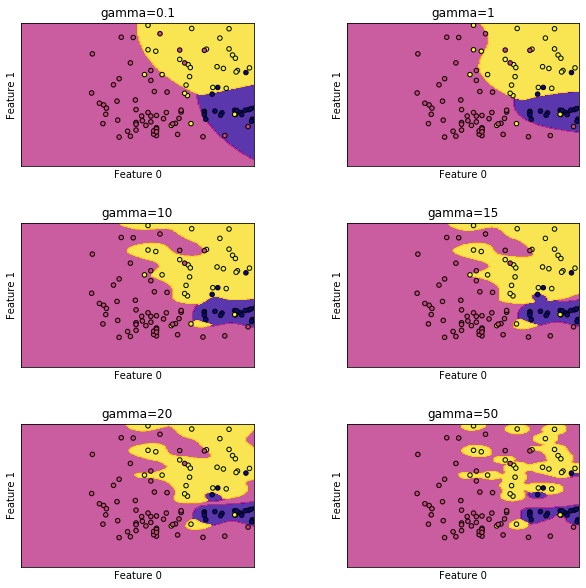【结果分析】

1. 随着gamma值的的增大，模型的非线性现象越来越明显。简单的说, 当gamma → 0时，模型越来越简单，对于训练样本错分的几率越来越大; 当gamma增大时，模型变得越来平滑、复杂，非线性现象也越来越明显，对于训练样本来说分错的几率也越来越小
2. 可以看到的是，当gamma变大时，测试集的性能并没有随着训练集性能的增加而持续增加，而是出现一个峰值后逐渐降低。
3. 总结一下：gamma值越小模型越来越趋近于欠拟合，gamma值越大模型越来越趋向于过拟合.

#### 2.2.2 多项式核的degree值¶

In :
# TODO: 1. 导入必须库 以及 定义必要的函数
import numpy as np
import matplotlib.pyplot as plt
# 导入 Wine酒 数据集
from sklearn import datasets
from sklearn.model_selection import train_test_split
# 导入支持向量机SVM
from sklearn import svm

# 定义一个绘图函数

def make_meshgrid(x, y, h=0.02):
x_min, x_max = x.min() - 1, x.max() - 1
y_min, y_max = y.min() - 1, y.max() - 1
xx, yy = np.meshgrid(np.arange(x_min, x_max, h),
np.arange(y_min, y_max, h))
return xx, yy

# 第一个一个绘制等高线的函数

def plot_contours(ax, clf, xx, yy, **params):
Z = clf.predict(np.c_[xx.ravel(), yy.ravel()])
Z = Z.reshape(xx.shape)
out = ax.contourf(xx, yy, Z, **params)
return out

# TODO: 2. 创建/导入数据

# TODO: 3. 数据预处理，包括训练集、测试集划分，数据正则化，数据清洗等
X = wine.data[:, :2]  # 为便于可视化仍然仅使用前两个特征
y = wine.target
X_train, X_test, y_train, y_test = train_test_split(X, y)

# TODO: 4. 构建模型，并进行模型训练（或称为拟合数据）
C = 1.0
models = (svm.SVC(kernel='poly', degree=1, C=C, gamma='auto'),
svm.SVC(kernel='poly', degree=2, C=C, gamma='auto'),
svm.SVC(kernel='poly', degree=3, C=C, gamma='auto'),
svm.SVC(kernel='poly', degree=4, C=C, gamma='auto'),
svm.SVC(kernel='poly', degree=5, C=C, gamma='auto'),
svm.SVC(kernel='poly', degree=6, C=C, gamma='auto'))
# TODO:考虑输出变量models
models = (clf.fit(X_train, y_train) for clf in models)

titles = ('degree=1',
'degree=2',
'degree=3',
'degree=4',
'degree=5',
'degree=6')

# TODO: 5. 输出模型准确率
# for model, title in zip(models, titles):
#     print("RBFSVM({0}): 训练集准确率: {1:.3f}, 测试集准确率: {2:.3f}".format(
#         title, clf.score(X_train, y_train), clf.score(X_test, y_test)))

# TODO: 6. 可视化分析
# 设定一个子图形的个数和排列方式
fig, sub = plt.subplots(3, 2, figsize=(10, 10))

# 使用自定义的函数进行画图
X0, X1 = X_train[:, 0], X_train[:, 1]
xx, yy = make_meshgrid(X0, X1)

for clf, title, ax in zip(models, titles, sub.flatten()):
plot_contours(ax, clf, xx, yy, cmap=plt.cm.plasma, alpha=0.8)
ax.scatter(X0, X1, c=y_train, cmap=plt.cm.plasma, s=20, edgecolors='k')
ax.set_xlim(xx.min(), xx.max())
ax.set_ylim(yy.min(), yy.max())
ax.set_xlabel('Feature 0')
ax.set_ylabel('Feature 1')
ax.set_xticks(())
ax.set_yticks(())
ax.set_title(title)

# 输出准确率
print("RBFSVM({0}): 训练集准确率: {1:.3f}, 测试集准确率: {2:.3f}".format(
title, clf.score(X_train, y_train), clf.score(X_test, y_test)))

plt.show()

RBFSVM(degree=1): 训练集准确率: 0.759, 测试集准确率: 0.889
RBFSVM(degree=2): 训练集准确率: 0.812, 测试集准确率: 0.889
RBFSVM(degree=3): 训练集准确率: 0.812, 测试集准确率: 0.889
RBFSVM(degree=4): 训练集准确率: 0.789, 测试集准确率: 0.867
RBFSVM(degree=5): 训练集准确率: 0.797, 测试集准确率: 0.867
RBFSVM(degree=6): 训练集准确率: 0.797, 测试集准确率: 0.822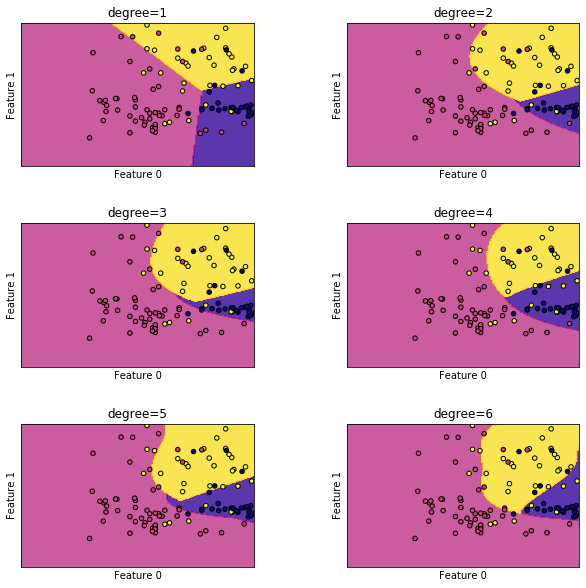【结果分析】

1. 随着degree值的的增大，模型的非线性现象越来越明显
2. 从现有设置来看，并未观察到模型准确率趋于稳定，原因可能是模型训练迭代次数不足

### 2.3 SVM算法的优缺点¶

1. 使用核函数可以向高维空间进行映射

2. 使用核函数可以解决非线性的分类

3. 分类思想很简单，就是将样本与决策面的间隔最大化

4. 分类效果较好

1. SVM算法对大规模训练样本难以实施（比如1万以上的样本，特别是特征较多的样本）

## 3. SVM实例——波士顿房价回归分析¶

SVM是重要的分类工具，它也可以用来做回归分析，下面我们使用sklearn数据集中非常适合做回归分析的波士顿房价数据集完成SVM回归分析。

### 3.1 数据集分析¶

• 载入必须库及数据集
In :
# TODO: 1. 导入必须库 以及 定义必要的函数
import matplotlib.pyplot as plt
# 导入 Boston波士顿房价数据集
from sklearn import datasets
from sklearn.model_selection import train_test_split
# 导入支持向量机SVM
from sklearn import svm
# 或使用 from sklearn.svm import SVR, 直接使用 SVR() 进行调用

# TODO: 2. 创建/导入数据

• 数据集分析
In :
# 预览数据集中的键
# 数据集中包含5个键，分别是数据、标签、特征名称、数据集描述和文件名
print(boston.keys())

dict_keys(['data', 'target', 'feature_names', 'DESCR', 'filename'])

In :
# 预览某个指定键的值
print(boston['filename'])

C:\Users\oxy\Anaconda3\lib\site-packages\sklearn\datasets\data\boston_house_prices.csv

In :
# 预览某个指定键的值
print(boston['DESCR'])

.. _boston_dataset:

Boston house prices dataset
---------------------------

**Data Set Characteristics:**

:Number of Instances: 506

:Number of Attributes: 13 numeric/categorical predictive. Median Value (attribute 14) is usually the target.

:Attribute Information (in order):
- CRIM     per capita crime rate by town
- ZN       proportion of residential land zoned for lots over 25,000 sq.ft.
- INDUS    proportion of non-retail business acres per town
- CHAS     Charles River dummy variable (= 1 if tract bounds river; 0 otherwise)
- NOX      nitric oxides concentration (parts per 10 million)
- RM       average number of rooms per dwelling
- AGE      proportion of owner-occupied units built prior to 1940
- DIS      weighted distances to five Boston employment centres
- TAX      full-value property-tax rate per $10,000 - PTRATIO pupil-teacher ratio by town - B 1000(Bk - 0.63)^2 where Bk is the proportion of blacks by town - LSTAT % lower status of the population - MEDV Median value of owner-occupied homes in$1000's

:Missing Attribute Values: None

:Creator: Harrison, D. and Rubinfeld, D.L.

This is a copy of UCI ML housing dataset.
https://archive.ics.uci.edu/ml/machine-learning-databases/housing/

This dataset was taken from the StatLib library which is maintained at Carnegie Mellon University.

The Boston house-price data of Harrison, D. and Rubinfeld, D.L. 'Hedonic
prices and the demand for clean air', J. Environ. Economics & Management,
vol.5, 81-102, 1978.   Used in Belsley, Kuh & Welsch, 'Regression diagnostics
...', Wiley, 1980.   N.B. Various transformations are used in the table on
pages 244-261 of the latter.

The Boston house-price data has been used in many machine learning papers that address regression
problems.

.. topic:: References

- Belsley, Kuh & Welsch, 'Regression diagnostics: Identifying Influential Data and Sources of Collinearity', Wiley, 1980. 244-261.
- Quinlan,R. (1993). Combining Instance-Based and Model-Based Learning. In Proceedings on the Tenth International Conference of Machine Learning, 236-243, University of Massachusetts, Amherst. Morgan Kaufmann.



### 3.2 数据集预处理¶

In :
# TODO: 3. 数据预处理，包括训练集、测试集划分，数据正则化，数据清洗等
X, y = boston.data, boston.target

X_train, X_test, y_train, y_test = train_test_split(X, y)


In :
print("训练集的形态: 数据{}, 标签{}".format(X_train.shape, y_train.shape))
print("训练集的形态: 数据{}, 标签{}".format(X_test.shape, y_test.shape))

训练集的形态: 数据(379, 13), 标签(379,)



### 3.2 使用SVR进行建模¶

In :
# TODO: 4. 构建模型，并进行模型训练（或称为拟合数据）
for kernel in ['linear','rbf', 'sigmoid']:
svr = svm.SVR(kernel=kernel, gamma='auto')
svr.fit(X_train, y_train)
print(kernel, '核函数的模型训练集得分:{:.3f}, 测试集得分:{:.3f}'.format(svr.score(X_train, y_train),svr.score(X_test, y_test)))

linear 核函数的模型训练集得分:0.704, 测试集得分:0.688
rbf 核函数的模型训练集得分:0.160, 测试集得分:-0.040
sigmoid 核函数的模型训练集得分:-0.015, 测试集得分:-0.060


1. SVM模型本身的性能有限
2. 样本各个特征之间存在较大的量级差异
3. 超参数设置不合理
4. ...

#### 3.3 模型优化¶

##### 3.3.1 利用正则化优化各个特征之间的量级差¶

In :
# 设置特征最小值的位置和标签
plt.plot(X.min(axis=0),'v',label='min')
# 设置特征最大值的位置和标签
plt.plot(X.max(axis=0),'^',label='max')
# 设置y轴采用对数形式显示
plt.yscale('log')
# 设置图例自动放置到最佳位置
plt.legend(loc='best')
# 设置坐标轴横轴、纵轴的标题
plt.xlabel('features')
plt.ylabel('feature magnitude')
# 显示图形
plt.show()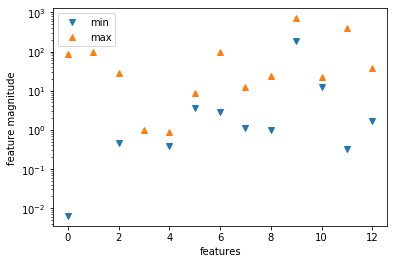【结果分析】

In :
# 导入数据预处理工具
from sklearn.preprocessing import StandardScaler

# 对训练集合测试集进行数据预处理：归一化
# 创建预处理训练器
scaler = StandardScaler()
# 提取训练集超参数
scaler.fit(X_train)
# 用预训练超参数拟合训练集和测试集
X_train_scaled = scaler.transform(X_train)
X_test_scaled = scaler.transform(X_test)

# 重新绘制特征最大值、最小值的位置和图标
plt.figure(dpi=120)
plt.plot(X_train_scaled.min(axis=0),'v',label='train set min')
plt.plot(X_train_scaled.max(axis=0),'^',label='train set max')
plt.plot(X_test_scaled.min(axis=0),'o',label='test set min')
plt.plot(X_test_scaled.max(axis=0),'*',label='test set max')
plt.yscale('log')
plt.legend(loc='best')
plt.xlabel('scaled features')
plt.ylabel('scaled feature magnitude')
plt.show()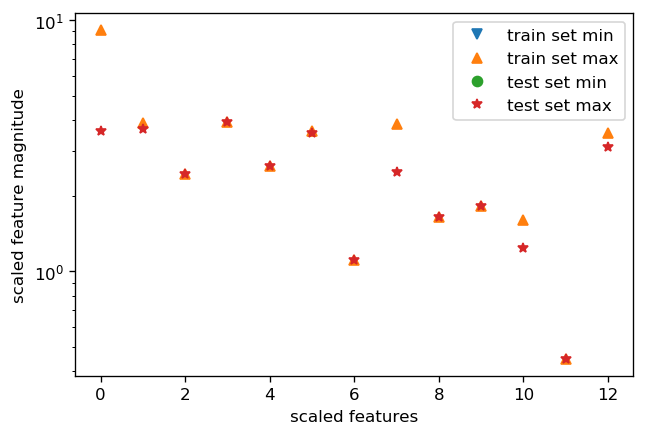In :
for kernel in ['linear','rbf', 'sigmoid']:
svr = svm.SVR(kernel=kernel)
svr.fit(X_train_scaled, y_train)
print('数据预处理后',kernel,'核函数的模型训练集得分: {:.3f}, 测试集得分：{:.3f}'.format(
svr.score(X_train_scaled, y_train), svr.score(X_test_scaled, y_test)))

数据预处理后 linear 核函数的模型训练集得分: 0.705, 测试集得分：0.680



【结果分析】

1. 从得分来看，无论是RBF核还是Sigmoid核，经过数据预处理后，无论是训练集还是测试集性能都有较大的提升
2. 线性核的SVM并没有太大的改善，说明归一化并不是对多有方法都有效果
3. 经过数据预处理后，RBF核和Sigmoid核都有显著提升，然而依然不如Linear核。这预示着，数据归一化后，模型仍然有提升的空间
##### 3.3.2 超参数调整¶

In :
kernel='rbf'
svr = svm.SVR(kernel=kernel, C=100, gamma=0.1)
svr.fit(X_train_scaled, y_train)
print('数据预处理后',kernel,'核函数的模型训练集得分: {:.3f}, 测试集得分：{:.3f}'.format(
svr.score(X_train_scaled, y_train), svr.score(X_test_scaled, y_test)))

数据预处理后 rbf 核函数的模型训练集得分: 0.957, 测试集得分：0.868


【本章小节】

1. SVM是目前使用比较广泛的机器学习算法，它不但可以实现二分类，也可以是实现多分类
2. SVM可以同时实现分类和回归分析
3. SVM最大的trick是核函数，常见的核函数包括线性核、高斯核(RBF)、Sigmoid核、多项式核等
4. SVM的性能提升主要来源于数据归一化和超参数调节。这也说明SVM对于数据和参数都较为敏感，这也使得要使SVM获得较好的性能需要丰富的调参经验。